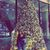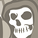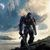Announcements
Meet our latest featured member Bruno! Drop a like and comment. READ ON
cancel
Showing results for
Did you mean:Contributor

## Compare two date dimensions using Set Analysis Expression

Hi All

I have all the columns as date columns instead of Invoice Amount column. I am trying to do the sum of Invoice Amount based on the below condition, but it is not working for me.

1st condition : I want to check Product Start Date and Sales Date is not null.

2nd condition : I want to check  Approval End Date less than Product Expiry Date.

Set Analysis Expression :-

Sum({\$< [ Product Start Date ]={"*"}, [Sales Date]={"*"},[Approval End Date] = {"<=\$(Date('[Product Expiry Date]', 'DD/MM/YYYY'))"}>}[Invoice Amount])

Sum I am getting is : 158,050.58 but Expected result is : 156,537.08

All Date formats are in (DD/MM/YYYY)

Would Appreciate for the help!:)

Labels (3)

• ### Scripting

11 RepliesCreator III

Hi,

Just to check your second condition:

2nd condition : I want to check Approval End Date less than Product Expiry Date.

But the logic in your set analysis is less than or equal to:

[Approval End Date] = {"<=\$(Date('[Product Expiry Date]', 'DD/MM/YYYY'))"}>}[Invoice Amount])Contributor
Author

Hi

It is less than equal to only . I forgot to write equal to in 2nd conditionMaster

In this case, creating a flag in the script would be an even better way.

If([Approval End Date] <= [Product Expiry Date], 1, 0) as Flag

and then this

``Sum({\$< [ Product Start Date ]={"*"}, [Sales Date]={"*"},Flag = {1}>}[Invoice Amount])``MVP

Right syntax for such field comparison would be like:

[Approval End Date] = {"=[Approval End Date]<=[Product Expiry Date]"}Contributor
Author

Approval End Date and Product Expiry Date are in different tables and there is no direct link between tables.Contributor
Author

Hi,

Above expression is giving me the less value than the expected result. It is not working.MVP

'giving less value'... might be becasue of some other issues but the set analysis syntax. Working of set analysis and IF structure is not same. It could be the case that you need IF, like below :

Sum({\$< [ Product Start Date ]={"*"}, [Sales Date]={"*"}>} If( [Approval End Date] <=[Product Expiry Date], [Invoice Amount]))

Try the above expression.Creator

I think this expression works for you.

Sum({\$< [ Product Start Date ]<>{"Null"}, [Sales Date]<>{"Null"},[Approval End Date] = {"<=\$(Date('[Product Expiry Date]', 'DD/MM/YYYY'))"}>}[Invoice Amount]).

Please like and accept the solution if u liked it.Contributor
Author

It is giving Sum as 0, not working.  Thank you 🙂Community Browser